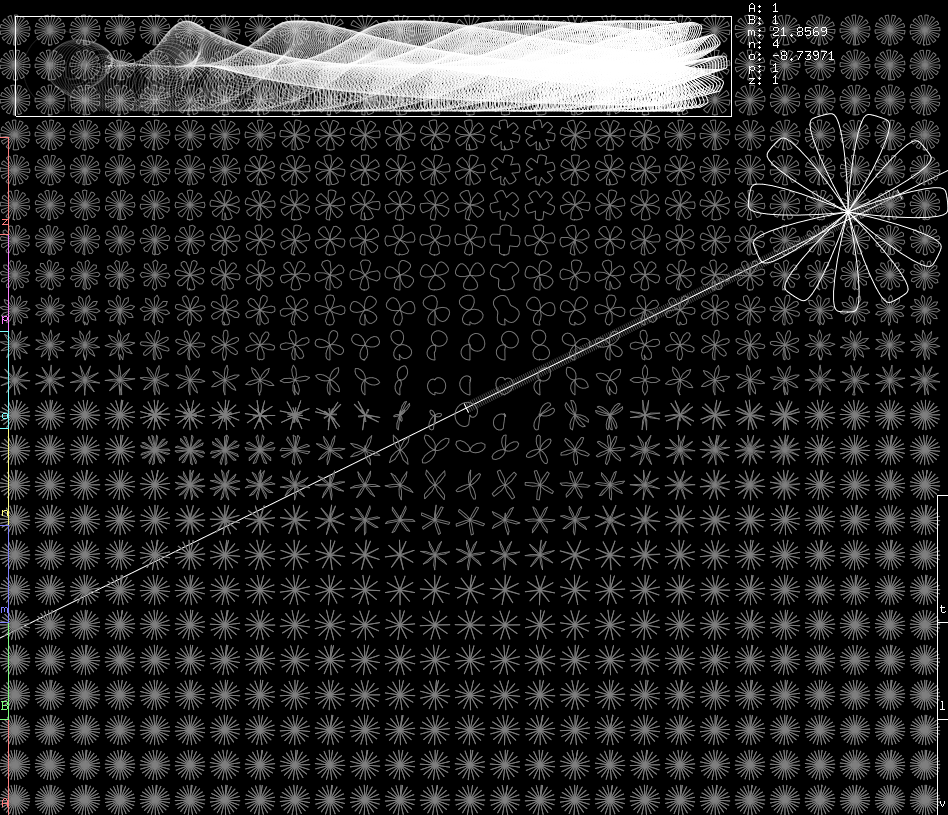# gte: Gielis Transform Explorergte stands for “Gielis Transform Explorer” and is an OpenGL interactive application that allows to explore the incredible variations of the Gielis Transform, also known as the Superformula.

Although other Superformula visualization tools exists, they all take the classical approach of assigning a slider to each variable and let you figure out how each parameter works.

gte instead is all about visual exploration. You can see the shapes varying in space, and at the same time define what space actually is. I designed gte when I met with Gielis himself around 2005-2007 in an attempt to come up with a different approach to multi-dimensional visualizations.

# The Superformula: a visual guide

In his original book Inventing The Circle Gielis shows the formula as follows:

r(φ) = |||(cos((mφ)/(4)))/(a)|||n + |||(sin((mφ)/(4)))/(b)|||o − (1)/(p) − z

This definition is slightly different than Wikipedia’s (we’ll discuss about the differences later), but what all those parameters actually mean?

If you wanted a mathematical introduction to the formula you could read MathWorld’s definition of the Superellipse.

TODO

# Implementation and UsageTODO